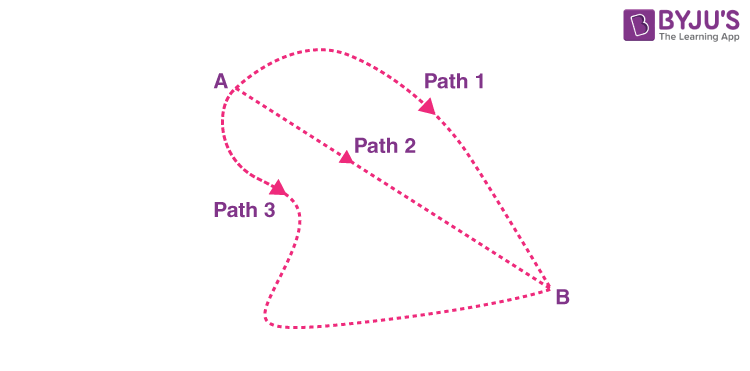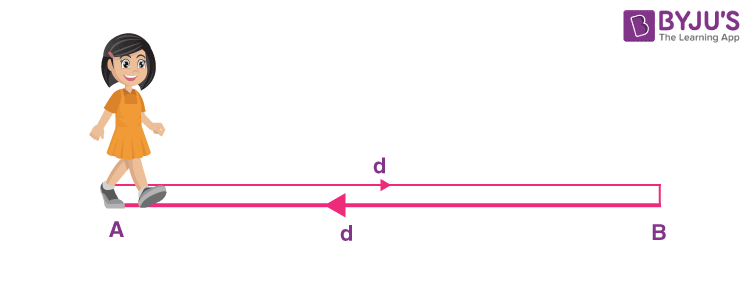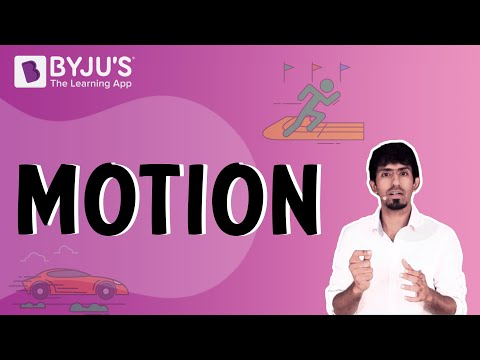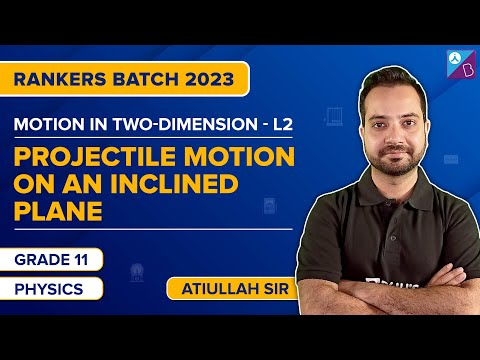# Introduction to Motion

When we talk about motion or rest, it is with reference to some point known as the origin. So, now with respect to the change in the position, we have two quantities which can be used to describe that change in position. They are distance and displacement. So now the question is, what is the difference between the two?
Talking about distance is defined as the total path length covered during the motion. It can be represented only by magnitude. On the other hand, displacement is the shortest distance between the initial and final positions. It requires both magnitude and direction for complete representation.

## What Is Motion?

We can define motion as the change of position of an object with respect to time. A book falling off a table, water flowing from the tap, rattling windows, etc., all exhibit motion. Even the air that we breathe exhibits motion! Everything in the universe moves. We live in a universe that is in continual motion. The fundamental particle of matter, that is, the atom, is in constant motion too. Every physical process in the universe is composed of motion of some sort. The motion can either be swift or slow, but motion exists. It is important that we give due attention to the study of motion because of its importance in the physical world. Motion is mainly described in terms of the following terms:

• Distance
• Displacement
• Speed
• TimeAs discussed earlier, distance and displacement are used to describe the change in position. Now, if someone asks what the distance between A and B is, we can’t give a definite answer because it depends on the path taken. It may or may not be the same for all three paths. But for displacement, we can always give a definite answer as it is a straight line joining the two points. In other words, displacement is nothing but the shortest distance between the two points, which in this case is Path 2. Also, it has a particular direction from A to B, as we can see.

So now that we have a basic idea of both, we will try to solve an example: Suppose the distance between two cities, A and B, is ‘d’. A person goes from A to B and returns. Calculate distance travelled and displacement.Distance travelled = Total path length covered

= d + d

= 2d

Displacement is measured as the shortest distance between the initial and final position. In this case, both are the same, and hence, displacement is also zero.

So for a motion, can displacement be greater than the distance covered? Think about it, and if not, can it be equal?

You may also want to check out these topics given below!

## The below video provides the Top 10 NTSE Important Questions on Motion Class 9## Types Of Motion

We might have noticed that different objects move differently. Some objects move in a curved path, some in a straight path and a few others in a different way. According to the nature of the movement, motion is classified into three types as follows:

• Linear Motion
• Rotary Motion
• Oscillatory Motion

### Linear Motion

In linear motion, the particles move from one point to another in either a straight line or a curved path. The linear motion depending on the path of motion, is further divided as follows

• Rectilinear Motion – The path of the motion is a straight line.
• Curvilinear Motion – The path of the motion is curved.

A few examples of linear motion are the motion of the train, football, the motion of a car on the road, etc.

### Rotatory Motion

Rotatory motion is the motion that occurs when a body rotates on its own axis.  A few examples of the rotatory motion are as follows:

• The motion of the earth about its own axis around the sun is an example of rotary motion.
• While driving a car, the motion of wheels and the steering wheel about its own axis is an example of rotatory motion.

### Oscillatory Motion

Oscillatory motion is the motion of a body about its mean position. A few examples of  oscillatory motion are

• When a child on a swing is pushed, the swing moves to and fro about its mean position.
• The pendulum of a clock exhibits oscillatory motion as it moves to and fro about its mean position.
• The string of the guitar when strummed moves to and fro by its mean position resulting in an oscillatory motion.

## Watch the Video and Learn about the Basic Concepts of Motion## Examples of Motion

Now let us understand motion clearly with the help of a few examples.

• Our daily activities, like walking, running, closing the door, etc. involve motion. There is a change of position of the object involved in these activities.
• The flow of air in and out of our lungs is also an example of motion.
• The automobiles that carry passengers from the place of pick up to the destination possess motion. In this case, the position of passengers is changed from one place to another.

## Frequently Asked Questions – FAQs

Q1

### State motion definition

As per motion definition, it is the change of position of an object with respect to time.
Q2

### What are the types of motion?

The following are the types of motion:

• Linear Motion
• Rotary Motion
• Oscillatory Motion
Q3

### What are the types of linear motion?

Types of linear motion are:
• Rectilinear Motion
• Curvilinear Motion
• Q4

TRUE
Q5

### Give some examples of motion

Examples of motion are:

• Walking
• Running
• The flow of air in and out of our lungs.
• The automobiles that carry passengers from the place of pick up to the destination.
• ## Watch the Video and Learn about 2D Motion and Projectile Motion## Watch the Video and Learn about Projectile Motion on Inclined PlaneStay tuned with BYJU’S to learn more about other Physics concepts with the help of interesting video lessons.

Test your knowledge on Introduction of motion

1. This motion is very interesting

2. This is great .motion a very simple subject now

3. thank you so much for helping me out for my project !

4. This helped to revise my concepts and also to correlate with everyday incidents!!proud to be a byjuite!!

5. It is very good.
I have any doubts I am clearing here only because it is very helpful and Teachers also chat and help us. Thank you!

6. motion was the toughest chapters later , but now after visiting this website i felt confident about dealing the subtopics .thankyou BYJUS.

7. Very interesting.
I liked it so much.